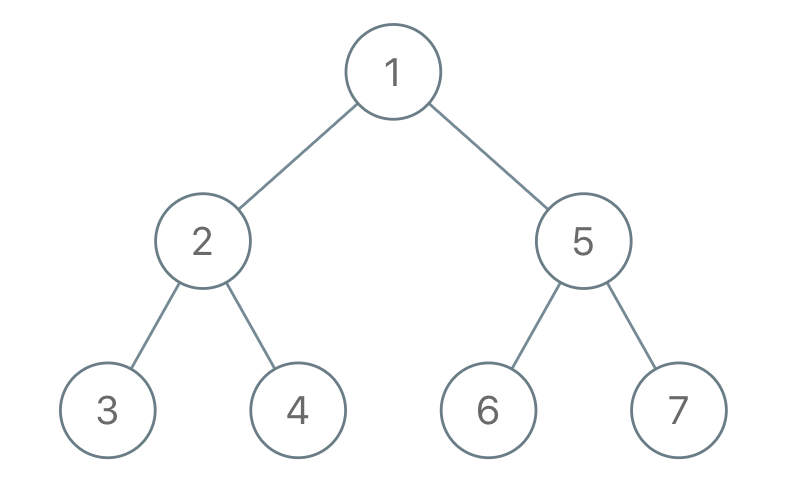# LeetCode Weekly Contest 132

https://leetcode.com/contest/weekly-contest-132

## Divisor Game

Alice and Bob take turns playing a game, with Alice starting first.

Initially, there is a number N on the chalkboard. On each player’s turn, that player makes a move consisting of:

• Choosing any x with 0 < x < N and N % x == 0.
• Replacing the number N on the chalkboard with N - x.

Also, if a player cannot make a move, they lose the game.

Return True if and only if Alice wins the game, assuming both players play optimally.

Example 1:

Example 2:

Note:

1. 1 <= N <= 1000

N=2时，一定能够使得对手面临上面的状况，那么就赢定了……

N为偶数时，一定有一个约数是奇数，减去奇数可以使得对手面临N为奇数的情况。而N为奇数时，所有约数都为奇数，轮到对手时就只可能是偶数了。也就是轮到奇数的玩家最终会一直面临N为奇数，直至最终到N=1一定会输，反之开局为偶数就会赢。

## Maximum Difference Between Node and Ancestor

Given the root of a binary tree, find the maximum value V for which there exists different nodes A and B where V = |A.val - B.val| and A is an ancestor of B.

(A node A is an ancestor of B if either: any child of A is equal to B, or any child of A is an ancestor of B.)

Example 1:Note:

1. The number of nodes in the tree is between 2 and 5000.
2. Each node will have value between 0 and 100000.

## Longest Arithmetic Sequence

Given an array A of integers, return the length of the longest arithmetic subsequence in A.

Recall that a subsequence of A is a list A[i_1], A[i_2], ..., A[i_k] with 0 <= i_1 < i_2 < ... < i_k <= A.length - 1, and that a sequence B is arithmetic if B[i+1] - B[i] are all the same value (for 0 <= i < B.length - 1).

Example 1:

Example 2:

Example 3:

Note:

1. 2 <= A.length <= 2000
2. 0 <= A[i] <= 10000

## Recover a Tree From Preorder Traversal

We run a preorder depth first search on the root of a binary tree.

At each node in this traversal, we output D dashes (where D is the depth of this node), then we output the value of this node. (If the depth of a node is D, the depth of its immediate child is D+1. The depth of the root node is 0.)

If a node has only one child, that child is guaranteed to be the left child.

Given the output S of this traversal, recover the tree and return its root.

Example 1:Example 2:Example 3:Note:

1. The number of nodes in the original tree is between 1 and 1000.
2. Each node will have a value between 1 and 10^9.